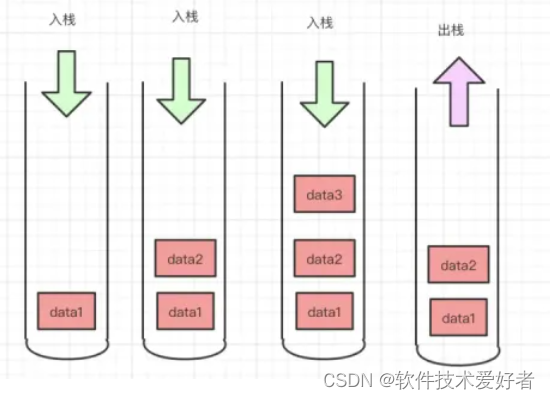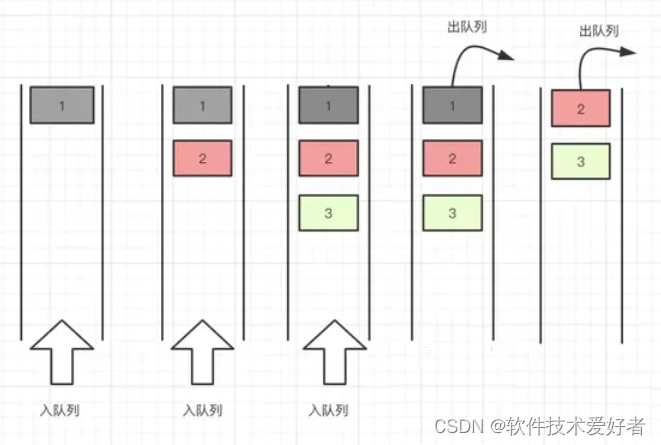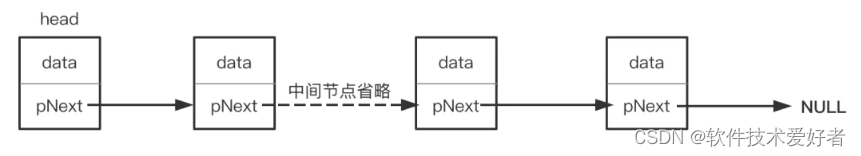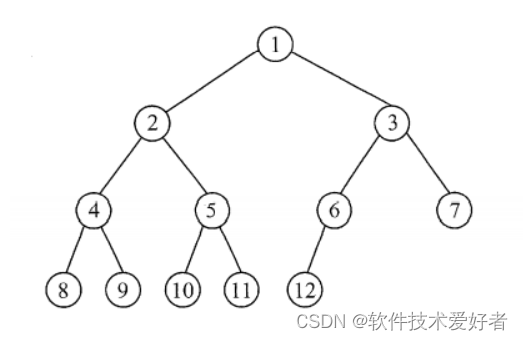## 使用Python实现几种底层技术的数据结构

news/2023/11/30 8:31:09

### 使用Python实现几种底层技术的数据结构

Python实现了许多常见的底层数据结构，以下是其中几种常见的：

★栈（Stack）``````class Stack:def __init__(self):self.items = []def is_empty(self):return not self.itemsdef push(self, item):self.items.append(item)def pop(self):if not self.is_empty():return self.items.pop()raise IndexError("pop from empty stack")def peek(self):if not self.is_empty():return self.items[-1]raise IndexError("peek from empty stack")def size(self):return len(self.items)#使用Stack类创建一个栈对象，并进行操作：
stack = Stack()
stack.push(1)
stack.push(2)
stack.push(3)
print(stack.size())    # 输出：3
print(stack.pop())     # 输出：3
print(stack.peek())    # 输出：2
print(stack.is_empty())     # 输出：False
``````

★队列（Queue）``````class Queue:def __init__(self):self.items = []def is_empty(self):return not self.itemsdef enqueue(self, item):self.items.insert(0, item)def dequeue(self):if not self.is_empty():return self.items.pop()raise IndexError("dequeue from empty queue")def size(self):return len(self.items)#使用Queue类创建一个队列对象，并进行操作：
queue = Queue()
queue.enqueue('a')
queue.enqueue('b')
queue.enqueue('c')
print(queue.size())    # 输出：3
print(queue.dequeue())     # 输出：'a'
print(queue.is_empty())    # 输出：False
``````下面是一个简单的示例，展示了如何在Python中实现单链表：

``````class Node:def __init__(self, data):self.data = dataself.next = Noneclass LinkedList:def __init__(self):self.head = Nonedef is_empty(self):return self.head is None        def append(self, data):if not self.head:self.head = Node(data)else:current = self.headwhile current.next:current = current.nextcurrent.next = Node(data)def display(self):elements = []current = self.headwhile current:elements.append(current.data)current = current.nextreturn elementsdef remove_node(self, data):if not self.is_empty():current_node = self.headif current_node.data == data:self.head = current_node.nextelse:while current_node.next:if current_node.next.data == data:current_node.next = current_node.next.nextbreakcurrent_node = current_node.nextdef get_size(self):size = 0current_node = self.headwhile current_node:size += 1current_node = current_node.nextreturn size#使用LinkedList类创建一个链表对象，并进行操作：
``````

★二叉树（Binary Tree）``````class TreeNode:def __init__(self, data):self.data = dataself.left = Noneself.right = Noneclass BinaryTree:def __init__(self, root):self.root = TreeNode(root)def insert_left(self, current_node, data):if current_node.left is None:current_node.left = TreeNode(data)else:new_node = TreeNode(data)new_node.left = current_node.leftcurrent_node.left = new_nodedef insert_right(self, current_node, data):if current_node.right is None:current_node.right = TreeNode(data)else:new_node = TreeNode(data)new_node.right = current_node.rightcurrent_node.right = new_node# 用于演示的简单遍历方法def preorder_traversal(self, start, traversal=[]):"""Root -> Left -> Right"""if start:traversal.append(start.data)self.preorder_traversal(start.left, traversal)self.preorder_traversal(start.right, traversal)return traversal#使用 BinaryTree类创建一个二叉树对象，并进行操作
# 创建一个二叉树对象
binary_tree = BinaryTree(1)# 插入左子节点
binary_tree.insert_left(binary_tree.root, 2)
# 插入右子节点
binary_tree.insert_right(binary_tree.root, 3)# 插入左子节点的左子节点
binary_tree.insert_left(binary_tree.root.left, 4)
# 插入左子节点的右子节点
binary_tree.insert_right(binary_tree.root.left, 5)# 插入右子节点的左子节点
binary_tree.insert_left(binary_tree.root.right, 6)
# 插入右子节点的右子节点
binary_tree.insert_right(binary_tree.root.right, 7)# 进行先序遍历
print(binary_tree.preorder_traversal(binary_tree.root))``````

### Thinkphp-商城项目之oss文件上传及web端直传

4.3头像上传 一般商城网站都会把文件上传到第三方云&#xff0c;例如阿里云(oss)&#xff0c;腾讯云(cos)&#xff0c;当然如果公司有足够的实力&#xff0c;可以自己部署一台文件服务器&#xff0c;用于文件的保存。 头像上传一般是用户在用户中心上传的&#xff0c;后台管理…

### 从0开始学习JavaScript--JavaScript使用Promise

JavaScript中的异步编程一直是开发中的重要话题。传统的回调函数带来了回调地狱和代码可读性的问题。为了解决这些问题&#xff0c;ES6引入了Promise&#xff0c;一种更现代、更灵活的异步编程解决方案。本文将深入探讨JavaScript中如何使用Promise&#xff0c;通过丰富的示例代…

### Oracle 11g 多数据库环境下的TDE设置

19c的TDE wallet的设置是在数据库中设置的&#xff0c;也就是粒度为数据库&#xff0c;因此不会有冲突。 而11g的设置是在sqlnet.ora中&#xff0c;因此有可能产生冲突。 这里先将一个重要概念&#xff0c;按照文档的说法&#xff0c;wallet是不能被数据库共享的。 If there …

### DES加密前端入参

js中使用DES加解密解决方案总结_js des加密-CSDN博客 1.需求背景 最近开发vue项目中&#xff0c;对于用户手机号码需要进行DES加解密操作。 简介&#xff1a;DES加密&#xff0c;是一种比较传统的加密方式&#xff0c;其加密运算、解密运算使用的是同样的密钥&#xff0c;信息…